## Three loops of wire, one circular, one rectangular, and one square, are made from identical lengths of wire. If the loops are placed in an i

Question

Three loops of wire, one circular, one rectangular, and one square, are made from identical lengths of wire. If the loops are placed in an increasing magnetic field perpendicular to the plane of the loops, which loop has the greatest induced emf? A) The circular one. B) The rectangular one. C) The square one. D) All three would have the same induced emf.

in progress 0
3 months 2021-07-25T02:31:19+00:00 1 Answers 4 views 0

A) The circular one

Explanation:

When there is a loop of wire in a magnetic field, and the magnetic flux through the coil changes, an electromotive force is induced in the coil, according to Faraday-Newmann-Lenz law: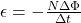where

N is the number of turns in the coil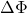is the change in magnetic flux through the coil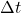is the time interval

The variation of flux through a coil can be written as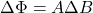where

A is the area of the coil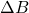is the change in magnetic field

Here we have three loops of wire: one circular, one rectangular, one square.

The length of the wire used for the 3 loops is the same, therefore their perimeter is also the same.

The change in magnetic flux is directly proportional to the area enclosed by the loop: therefore, the loop that will experience the greatest induced emf is the one having the greatest area.

Since the circle is the 2D figure that maximizes the area for a given perimeter, this means that the circular loop has the greatest area, and so also the greatest induced emf.